# How to Subtract Fractions with Unlike Denominators

How to Subtract Fractions with Unlike Denominators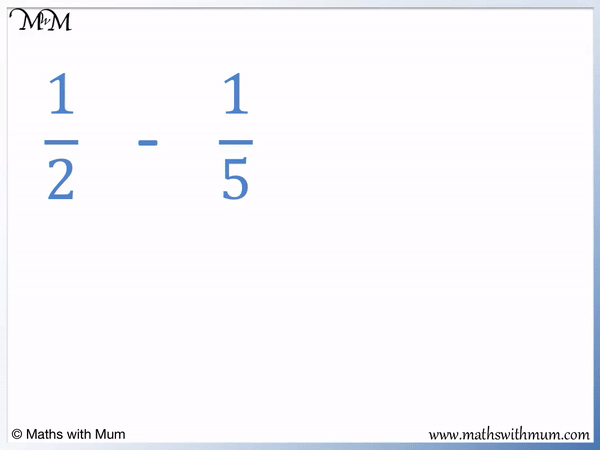• To subtract fractions, they must first have the same denominator on the bottom.
• Find the first number that is in the times tables of each denominator. We call this the least common denominator.
• The first number in both the 2 and 5 times table is 10. 10 is the least common denominator.
• Write each fraction out of this least common denominator using equivalent fractions.
•   1/2   can be written as   5/10   by multiplying the numbers by 5.
•   1/5   can be written as   2/10   by multiplying the numbers by 2.
• Once the denominators are the same, the fractions can be subtracted.
• Keep the denominator on the bottom the same and subtract the numerators on top.
•   5/10   -   2/10   =   3/10   and therefore   1/2   -   1/5   =   3/10  .
Convert the fractions to like fractions with the same denominator before subtracting them.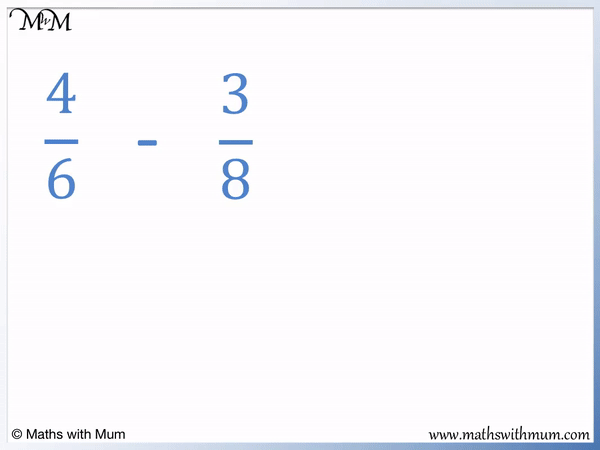• To subtract fractions with different denominators, first write them as equivalent fractions with the same denominator.
• The denominators of 6 and 8 have a least common denominator of 24. This means that 24 is the first number in both the 6 and 8 times table.
• 4/6   can be written as   16/24   by multiplying the values by 4.
• 3/8   can be written as   9/24   by multiplying the values by 3.
• 16/24   -   9/24   =   7/24  .
• Therefore   4/6   -   3/8   =   7/24  .Supporting Lessons

#### Subtracting Fractions with Unlike Denominators# Subtracting Fractions with Unlike Denominators

## How to Subtract Fractions with Unlike Denominators

To subtract fractions with unlike denominators, follow these steps:

1. Find the least common multiple of the denominators of the fractions.
2. Write equivalent fractions that have the least common multiple as the denominator.
3. Keep this least common multiple as the denominator of the answer.
4. Subtract the numerators in the question to get the numerator of the answer.

The most important rule when subtracting fractions is to first make a common denominator. The denominators of the fractions must be the same before subtracting them.

For example, here is   4/6   -   3/8  .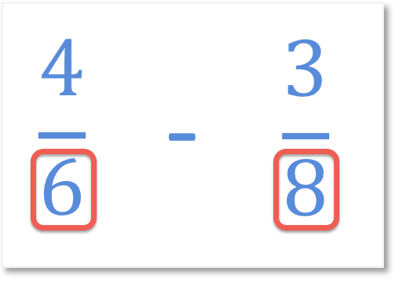Denominators are the numbers on the bottom of the fraction. The denominators are 6 and 8, which are different. To subtract fractions, we need the denominators to be the same.

A common denominator is a number that is a multiple of all of the other denominators. It is usually chosen as the first number that appears in all of the times tables of the denominators. For example, with   4/6   -   3/8  , the common denominator is 24 because 24 is the first number in the 6 and 8 times tables.To find the least common denominator, list the multiples of each denominator and write down the first number to appear in every list. Alternatively, a common denominator can be found by multiplying the denominators together.

The multiples of 6 are 6, 12, 18, 24 and the multiples of 8 are 8, 16, 24. 24 is the first number to appear in each list and so, it is the least common denominator of 6 and 8. The least common denominator is also commonly known as the lowest common denominator. It is the smallest common denominator that can be found.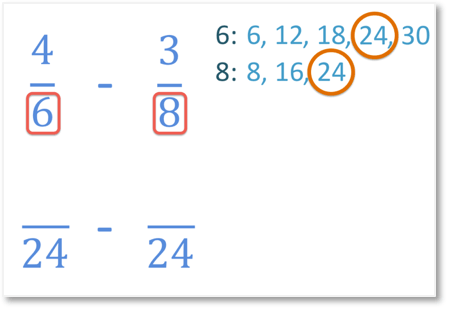The next step is to find equivalent fractions that have the least common denominator.

The first fraction denominator has been multiplied by 4. Therefore we need to multiply the numerator by 4 as well.

The denominator calculation is 6 × 4 = 24. The numerator calculation is 4 × 4 = 16. Both the numerator and denominator are multiplied by 4.The second fraction denominator has been multiplied by 3. Therefore we need to multiply the numerator by 3 as well.

The denominator calculation is 8 × 3 = 24. The numerator calculation is 3 × 3 = 9. Both the numerator and denominator are multiplied by 3.Now that the fractions have common denominators, the subtraction can be done. The denominator of the answer is the same as the common denominator.

The lowest common denominator is 24 and so, the denominator of the answer is also 24.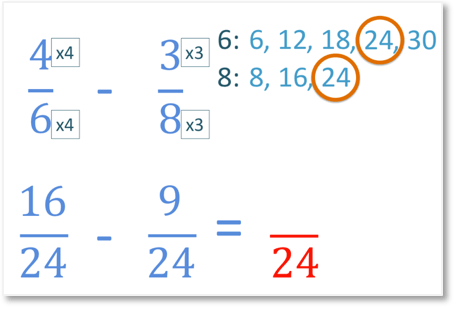To find the numerator of the answer, simply subtract the numerators in the question.

16 - 9 = 7 and so, the numerator of the answer is 7.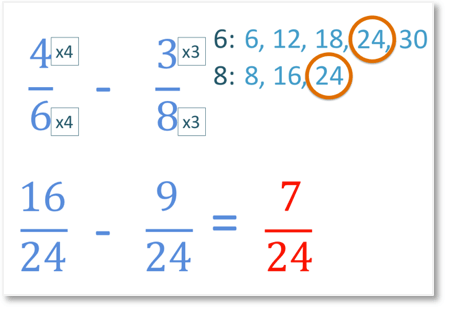4/6   -   3/8   =   7/24  .Here is another example of subtracting unlike fractions step by step.

We have   1/2   -   1/5  .

The denominators are different and so, we need to find equivalent fractions with a common denominator.The multiples of 2 are 2, 4, 6, 8, 10 and the multiples of 5 are 5 and 10.

We can see that 10 is the first number in both lists and so, 10 is the least common denominator of 2 and 5.

We write   1/2   as   5/10  .

We write   1/5   as   2/10  .

Now that we have a common denominator, we can subtract the fractions   5/10   -   2/10   =   3/10  .

If both denominators are prime, the least common denominator is found by multiplying the denominators together. For example in   1/2   -   1/5  , the least common denominator is 10 because 2 × 5 = 10.

## Subtracting Fractions with the Butterfly Method

The butterfly method is a short method that can be used for adding or subtracting 2 fractions. It involves multiplying the numerator of one fraction by the denominator of the other with the bubbles around each multiplication drawn to make an image of a butterfly.

To subtract fractions using the butterfly method, follow these steps:

1. Multiply the two denominators together to find the denominator of the answer.
2. Multiply the first numerator by the second denominator.
3. Multiply the second numerator by the first denominator.
4. Write both of these two answers on the numerator, separated by a subtraction sign.
5. Work out the subtraction to get one number as the numerator.
6. Simplify the fraction if possible.

For example, we have   4/5   -   2/3  .

The diagram below shows how the butterfly method works.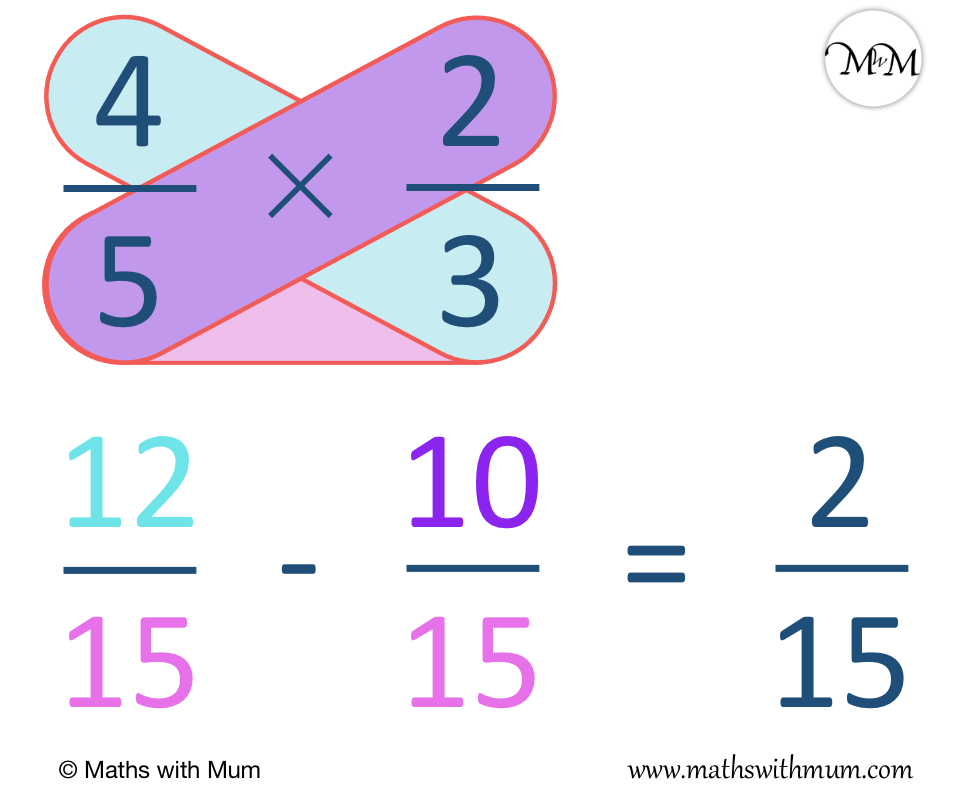We first multiply the denominators of 5 and 3.

5 × 3 = 15 and so the denominator of the answer is 15.

Next we multiply the numerator of the first fraction by the denominator of the second fraction.

4 × 3 = 12, so we write 12 on the numerator of the fraction.

Next we multiply the numerator of the second fraction by the denominator of the first fraction.

2 × 5 = 10 and so we write a 10 alongside the 12 with a subtraction sign in between.

For each multiplication in the butterfly method, draw a bubble around the numbers. This makes the overall calculation look like a butterfly and can help make the method easier to remember and learn.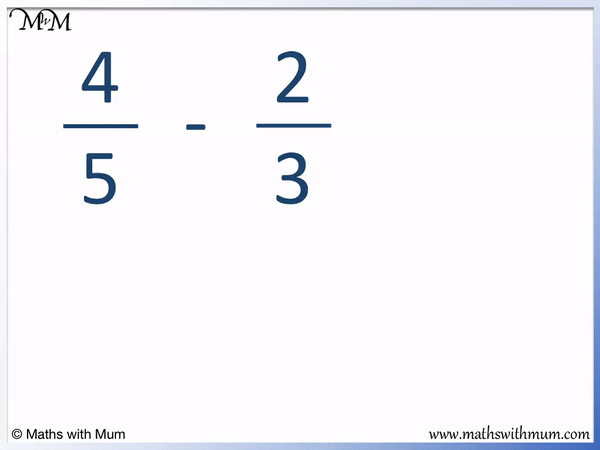Finally, we work out the subtraction on the numerator.

12 - 10 = 2 and so, 2 is the numerator.

The result of the butterfly method calculation is   2/15

The butterfly method is an easy way to routinely solve the addition and subtraction of 2 fractions. The benefits of the butterfly method are that it reduces working out and the method is easier to remember due to the symmetrical butterfly pattern. It is a useful method to teach when the initial understanding of how to add and subtract fractions has been learnt.

The main problem with the butterfly method is that it only allows for the addition and subtraction of two fractions. It is not recommended to introduce adding and subtracting fractions with the butterfly method because it does not allow for a strong understanding of why the method works and it is limited to use on specific types of question.

## Subtracing Unlike Fractions Easy Examples

Here are some easier examples to practise with. When teaching subtracting fractions with unlike denominations, it is helpful to start with examples where only one fraction needs changing.

These examples only require one fraction to change in order to find a common denominator. If one fraction denominator is a multiple of the other, only one fraction needs to be changed.

The first easy example is   1/2   -   1/4  .

We can see that the denominator of 4 is a multiple of the denominator of 2.

This means that we can simply double the values in the fraction   1/2   to get a common denominator of 4.   1/2   =   2/4  .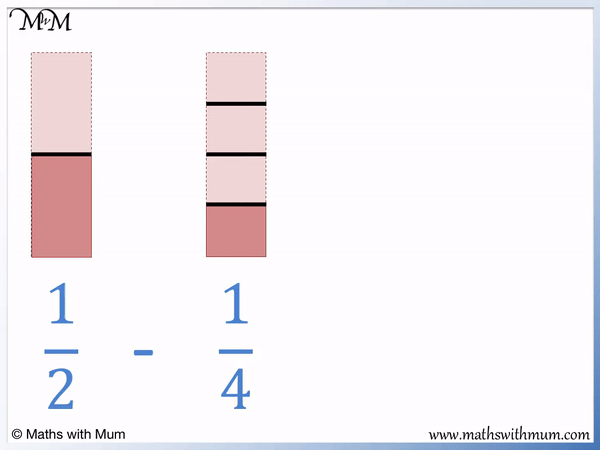We rewrite   1/2   -   1/4   as   2/4   -   1/4  .

2 - 1 = 1 and so, the numerator of the answer is 1. The denominator remains as 4.

Here is another easy example of subtracting fractions with different denominators.

We have   11/12   -   3/4  .

We can see that 12 is a multiple of 4 and so, only one fraction needs changing. The 4 needs to be multiplied by 3 to make 12.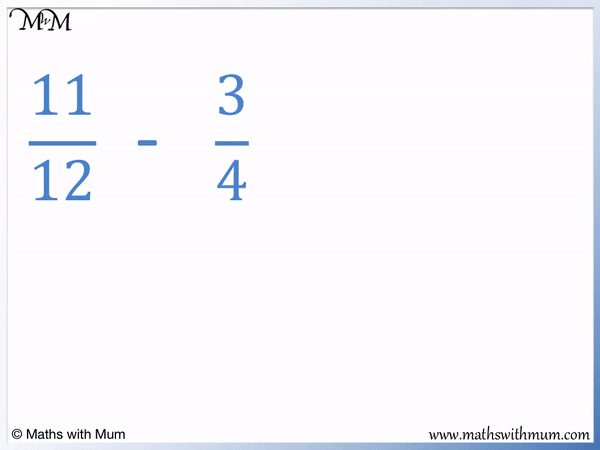We rewrite the fraction   3/4   as   9/12  .

11/12   -   9/12   =   2/12  .

It is possible to simplify this answer by halving both the numerator and denominator.

2/12   simplifies to   1/6  .

## How to Subtract Mixed Numbers with Unlike Denominators

To subtract mixed numbers with unlike denominators, follow these steps:

1. Write the mixed numbers as improper fractions.
2. Find the least common denominator.
3. Write the improper fractions as equivalent fractions that have the least common denominator.
4. The denominator of the answer is the same as this least common denominator.
5. Subtract the numerators to find the numerator of the answer.

For example, here is 5   1/4   - 2   2/3  .

The first step is to convert the mixed numbers into improper fractions.

To convert a mixed number to an improper fraction, multiply the whole number by the denominator and add the numerator. This result is the new numerator and the denominator is the same as the denominator of the mixed number.

5 × 4 = 20 and then 20 + 1 = 21.

The mixed number of 5   1/4   can be rewritten as an improper fraction as   21/4  .

2 × 3 = 6 and then 6 + 2 = 8.

The mixed number of 2   2/3   can be rewritten as an improper fraction as   8/3  .Now that the mixed numbers have been written as improper fractions, the next step in the subtraction is to find the lowest common denominator.

The first number in both the 4 and 3 times table is 12. The least common denominator is 12.

We multiply the denominator and numerator of the first fraction by 3 and the second fraction by 4.

21/4   is rewritten as   63/12  .

8/3   is rewritten as   32/12  .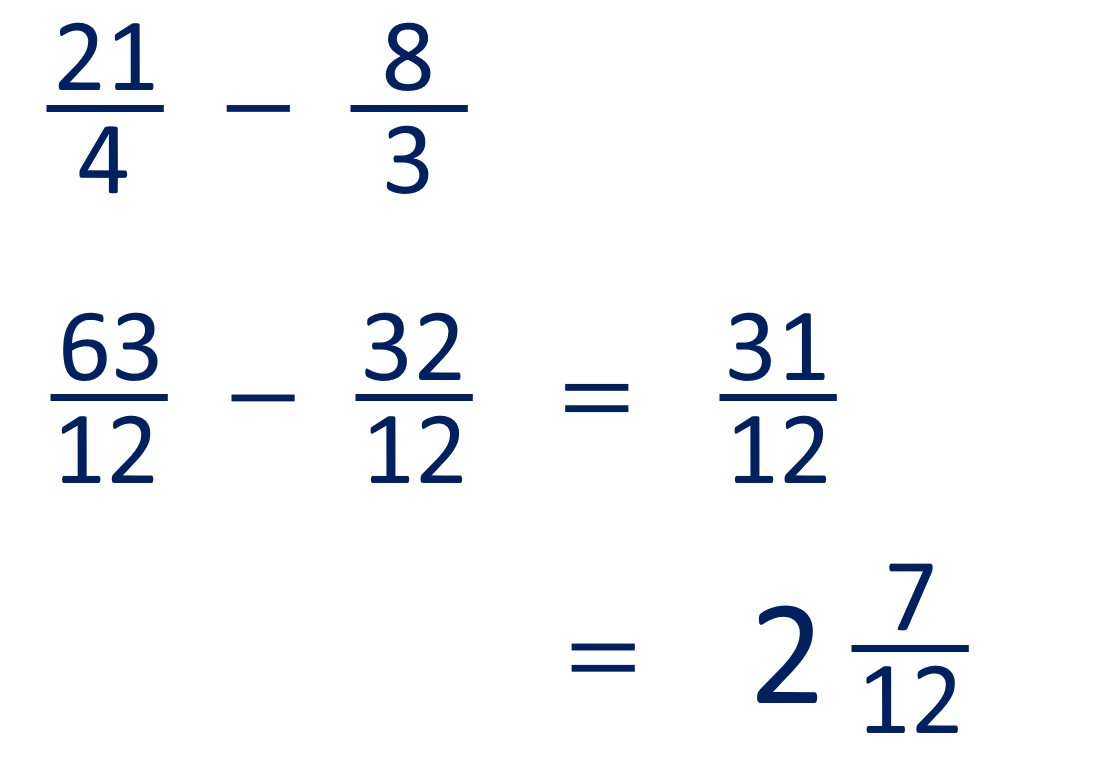Now that the mixed numbers have been converted into improper fractions and the improper fractions now have common denominators, we can finally perform the subtraction.

The denominator remains the same and we subtract the numerators.

63/12   -   32/12   =   31/12  .

The final step is to write the improper fraction as a mixed number if necessary.

31 ÷ 12 = 2 remainder 7. We write the whole number down and the remainder as the numerator of the fraction.

The improper fraction of   31/12   is written as the mixed number of 2   7/12  .